# Difference between revisions of "SMHS LinearModeling LMM"

## SMHS Linear Modeling - Linear Mixed Effects Analyses

Scientific inference based on fixed and random effect models, assumptions, and mixed effects logistic regression.

Questions:

• What happens if data are not independent and identically distributed (IIDs)?
• How to model multiple observations for the case/subject (across time, conditions, etc.)?

### Fixed and Random Effects

Linear models express relationships between data elements (variables) in terms of a (linear) function. For example, we can model weight as a function of height.

Weight ~ Height + ε

Here, “height” is a fixed effect, and ε is our “error term” representing the deviations between the model predictions and observations (of weight) due to “random” factors that we cannot control experimentally. This tem (ε) is the “probabilistic” or “stochastic” part of the model. Let’s try to unpack “ε” and add complexity to it. In mixed (fixed and random effect) models, everything in the “systematic” part of your model works just like with linear models. If we change the random aspect of our model this leaves the systematic part (height) unchanged.

Suppose we’re looking at the Baseball data and try to identify a relationship that looks like this:

Weight ~ position + ε

Position (player field position) is treated as a categorical factor with several levels (e.g., Catcher, First-Baseman, etc.) On top of that, we also have an additional fixed effect, Height, and so our bivariate linear model looks more like this:

Weight ~ Height + position + ε

This model expansion is nice, but it complicates a bit the data analytics and scientific inference. If the study design involved taking multiple measures per player, say across time/age, each player would yield multiple position, height and weight responses. According to the assumptions of the linear model, this would violate the independence assumption, as multiple responses from the same subject cannot be regarded as independent from one another. Every player has a slightly different weight, and this is going to be an idiosyncratic factor that affects all responses from the same player, thus rendering these different responses inter-dependent (within player) instead of independent, as required by the model assumptions.

A way to resolve this model assumption violation is to add a random effect for players. This allows us to account for inter-independences by assuming a different “baseline” weight value for each player. For example, player 1 may have a mean weight 200 pounds across different times, and player 2 may have a mean weight of 350 pounds. Here’s a visual depiction of how this looks like:

 Team A_Player1 Team A_Playe2 Team A_Player3 Team A_Player4 Team A_Player5 Team B_Player1 Team B_Player2 Team B_Player 3 160 340 240 340 180 200 240 180 180 340 240 400 240 200 320 120 220 320 320 400 260 180 340 160 240 300 300 380 320 160 300 160 200 380 300 380 280 260 300 260 220 360 280 360 280 180 300 180 260 360 320 400 260 240 320 180 200 360 280 380 300 220 320 200 220 480 260 380 280 180 300 220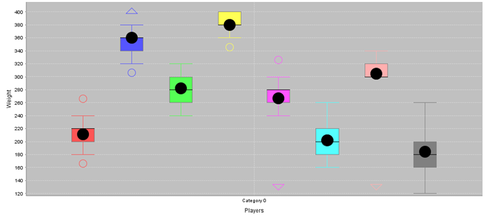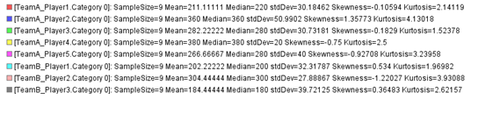SOCR Charts generated these plots (http://www.socr.umich.edu/html/cha/). The same data may similarly be plotted using R:

data <- read.table('C:\\Users\\Dinov\\Desktop\\01a_data.txt',as.is=T, header=T)
attach(data)
boxplot(TeamA_Player1, TeamA_Player2, TeamA_Player3, TeamA_Player4, TeamA_Player5, TeamB_Player1, TeamB_Player2, TeamB_Player3, col=c("white","lightgray"))
boxplot(data, las = 2)
boxplot(data, las = 2, par(mar = c(8, 5, 4, 2)+ 0.1))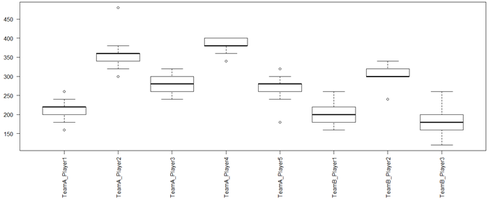We can model these individual differences by assuming different random intercepts for each player. In other words, each player will be assigned a different intercept value, and the mixed model estimates these intercept values.

The (fixed effects) linear models include several fixed effects and a general error term “ε”. Linear modeling segregates the world into things that we understand as systematic, i.e., fixed effects or the explanatory variables, and things that we cannot control for or poorly understand (error, ε). Statistical inference requires that the unsystematic part (ε) of the model does not have any interesting structure or pattern – it should represent random white noise with common (iid) across-the-board characteristics.

In mixed modeling, we add one or more terms to the fixed effects to account for random effects. These random effects essentially generalize the model (make it more applicable in situations when the error term does have structure) – that is, the random effect models pull out structure from the error term “ε”. For instance, in the baseball weights example, we add a random effect for “player”, and this characterizes idiosyncratic variation that is due to individual body build differences. The intertwining of fixed and random effects is what makes these models mixed-effect models.

Our updated formula looks like this:

M1: Weight ~ Height + position + ε1
M2: Weight ~ Height + position + (1|player) + ε2

“(1|player)” is the R notation for random player effects. It assumes an intercept that’s different for each player”, and “1” stands for the constant-term or intercept. This formula explicitly states that the model should account for (inter-player dependencies) multiple responses per player (say over time) – i.e., account for different baselines for each player. This effectively resolves the conflict with weight-dependences within players that stem from multiple weight observations.

The mixed effect model still contains a general error term “ε”, because although it accounts for individual by-player variation, there are still going to be “random” differences between different body-size measurements (e.g., weight) from the same player (noise).

The player position represents an additional source of non-independence that may need to be accounted for. Similarly to the case of by-player variation, we may expect by-position variation. For instance, there might be some special body-size demands for different positions that may lead to overall higher/lower weight. The weight measurements for different players may be similarly affected by this random factor due to game-position-specific idiosyncrasies. Thus, the different responses to one position may not always be independent. There may be some similarities in multiple weight measurements for the same play-position – even if these come from different players. Disregarding these interdependencies, we may violate the linear model independence assumptions. Below is an exemplary visual representation of the by-position variability in weight.

data <- read.table('https://umich.instructure.com/files/330381/download?download_frd=1&verifier=HpfmjfMFaMsk7rIpfPx0tmz960oTW7JA8ZonGvVC',as.is=T, header=T)
library("reshape2")
# melting by "Position". melt is from the reshape2 package.
# do ?melt for help
data.m <- melt(data[,-c(1:2)], id.var = "Position")
#
require(ggplot2)
ggplot(data = data.m, aes(x=variable, y=value)) + geom_boxplot(aes(fill=Position))

ggplot(data = data.m, aes(x=Position, y=value)) +
geom_boxplot() + facet_wrap(~variable,ncol = 4)

p <- ggplot(data = data.m, aes(x=variable, y=value))
p <- p + geom_boxplot(aes(fill = Position))
# to color the points replace group with color=Position
p <- p + geom_point(aes(y=value, group=Position), position = position_dodge(width=0.75))
p <- p + facet_wrap( ~ variable, scales="free")
p <- p + xlab("x-axis") + ylab("y-axis") + ggtitle("Title")
p <- p + guides(fill=guide_legend(title="Legend_Title"))
p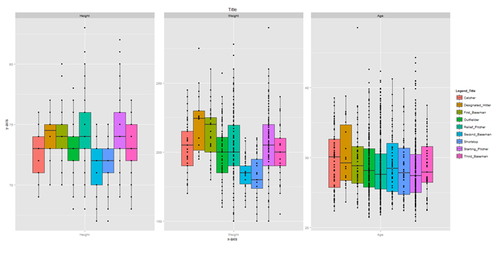The variation between positions may be different from the variation between players!

To add an additional random effect for position we expand the model:

Weight ~ Height + position + (1|player) + (1|position) + ε

Note that a similar argument may be made for “Team”, there could be error-term dependencies or patterns reflecting the between-team random effects on weight – some teams may be bulkier than others. This is really well documented in sports (e.g., top notch Serbian and Spanish water polo teams have a vastly different physiques).

data.m2 <- melt(data[,-c(1,3)], id.var = "Team")
# require(ggplot2)
p <- ggplot(data = data.m2, aes(x=variable, y=value)) + geom_boxplot(aes(fill=Team))
p + facet_wrap( ~ variable, scales="free")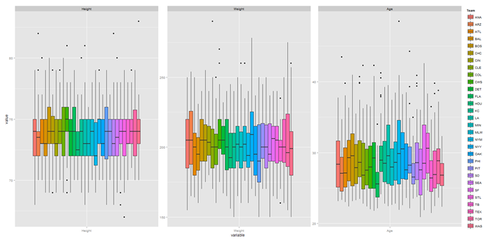Thus, in addition to modeling different intercepts for different players, we may include, as necessary, different intercepts for different positions or teams. This “resolves” dependencies in the linear model, potentially accounting for multiple responses per player, position or team, and we correctly representing the model by-player and by-position variation in overall weight.

Prior to the advent of mixed linear models, researchers used the averages of all multiple records. For example, in nursing, researchers may average the vital signs (e.g., heart rates, temperatures, etc.) of their patients over time or condition for a patient-based analysis where each data point is assumed to be derived from one subject, assuring independence. Then researchers may also average over subjects for a diagnostic condition type analysis, where each data point comes from one condition. There are advantages and disadvantages of this averaging approach. Whereas traditional analyses based on averaging are in principle correct, mixed models provide more flexibility and take the complete data into account. In a patient-based analysis (averaging over conditions/Dx), we basically disregard the by-condition variability. Conversely, in a condition-based analysis, we may disregard by-patient variation. A mixed-effect model would account for both sources of variation in a single analysis.

Let’s apply this understanding of the linear mixed effects modeling via R.

R commands for mixed-effect modeling (See appendix for complete R script) Open RStudio9 and install the R package lme410  :

install.packages(“lme4”)
library(lme4)


This makes available the function lmer(), which is the mixed model equivalent of the function lm() in the fixed-effect model. Load some data in RStudio11,12 .

data = read.csv(file.choose( ))
attach(data)


You can also try the R QCC package (for quality control, see the first section of this tutorial):

install.packages("qcc")
library("qcc", lib.loc="~/R/win-library/3.1")
data(orangejuice)
attach(orangejuice)
qcc(D[trial], sizes=size[trial], type="p")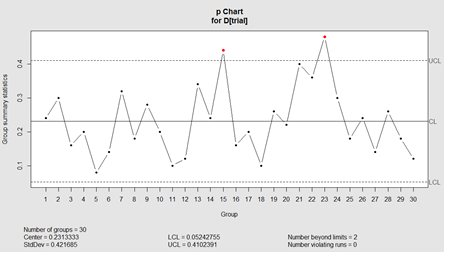Test the data-import and get a summary by using head(), tail(), summary(), str(), colnames(), or whatever commands you commonly use to get an overview of a dataset. Also, it is always good to check for missing values:

which(is.na(Team)==T)

If there are missing values, there are no problems for the mixed-effect modeling.

Using the MLB Baseball data13 we can explore relationship between variables (e.g., Height and Weight) by means of boxplots:

# data <- read.table('E:\\Ivo.dir\\Research\\UMichigan\\Education_Teaching_Curricula\\2015_2016\\HS_853_Fall_2015\\Modules_docx\\data\\01a_data.txt',as.is=T, header=T)
boxplot(Weight ~ Height, col=c("white","lightgray"))
boxplot(Weight ~ Height*team, col=c("white","lightgray"))Is the thick median line in the middle of the boxplot lower for the light than the heavier players?

To construct the mixed model try the command below …

lmer(Weight ~ Height, data=data)


You will get an error that should look like this:

Error: No random effects terms specified in formula


This is because the model needs at least 1 random effect however we only specified a single fixed effect (Height). So, let’s add random intercepts for subjects and items (remember that items are called “scenarios” here):

model.lmer <- lmer(Weight ~ Height + (1|Team) + (1|Position), data= data)


This command creates a model that includes a fixed effect “Height” to predict Weight, controlling for by-team and by-position variability. The model is saved in an object model.lmer. To see the model type in model.lmer – this will print the output in the shell. Note that in the classical fixed effect model, lm(), we need to use summary() to get this output.

This is the full output:

summary(model.lmer)

Linear mixed model fit by REML ['lmerMod']
Formula: Weight ~ Height + (1 | Team) + (1 | Position)
Data: data

REML criterion at convergence: 8841.4

Scaled residuals:
Min      1Q   Median      3Q     Max
-2.9396 -0.6885 -0.0222  0.6010  4.1358

Random effects:
Groups   Name      Variance   Std.Dev.
Team    (Intercept)   1.285   1.133
Position (Intercept)  56.218   7.498
Residual             295.652  17.195
Number of obs: 1034, groups:  Team, 30; Position, 9

Fixed effects:
Estimate Std. Error t value
(Intercept) -142.8215    19.0160  -7.511
Height         4.6973     0.2571  18.271

Correlation of Fixed Effects:
(Intr)
Height -0.991


The output starts with the user-specified model and data. Then, there’s the restricted maximum likelihood (ReML) criterion, summary of the model residuals (ε), followed by random and fixed effects.

The standard deviation column represents a measure of the variability for each random effect included in the model. “Team” has much less variability than Position. The Residual row represents the variability that’s not due to either Team or Position. This is the ε term – random deviations from the predicted values that are not due to Team or Position. This reflects the fact that body-size has some factors that affect Weight that are outside of the observations we have in this experiment. Similarly, to the fixed effect models, the fixed effects output in this mixed model output includes estimates, SE and T-statistic.

The coefficient of “Height” (4.6973) is the slope for this variable. Finally, the Correlation of the Fixed Effects (H vs. Intercept) is reported to be negative! The output under "correlation of fixed effects" has a different interpretation from the intuitive meaning. It is not about the correlation of the variables, but about the “expected correlation of the regression coefficients”. This may, or may not, be related to multicollinearity. In this case, corr(H,Intrc)= -0.991 suggests that if we redo the experiment again a decrease of the Height coefficient will increase the intercept, and vice-versa, an increase of the Height coefficient is expected to drive down the intercept term (which is currently =-142.8215).

plot(Height, Weight, main="Scatterplot Height vs. Weight", xlab="Height ", ylab="Weight ", pch=19)
abline(lm(Weight~Height), col="red") # regression line (W~H)
lines(lowess(Height,Weight), col="blue") # lowess line (H,W), (locally weighted scatterplot smoothing)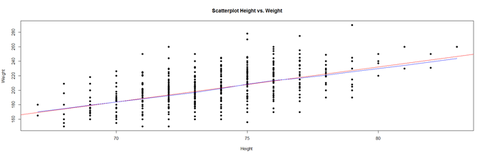Inference on LMER coefficients: The Satterthwaite approximation, implemented in the lmerTest package, overloads the lmerM function, using exactly the same model, but the summary() will include approximate degrees of freedom and p-values for all predictors.

# install.packages("lmerTest")
library(lmerTest)

# re-fit model
model.lmer <- lmer(Weight ~ Height + (1|Team) + (1|Position), data= data, REML = FALSE)
coeffs <- data.frame(coef(summary(model.lmer)))

# get Satterthwaite-approximated degrees of freedom
coeffs$\$$df.Satt <- coef(summary(model.lmer))[, 3] # get approximate p-values coeffs\$$p.Satt <- coef(summary(model.lmer))[, 5] coeffs Estimate Std..Error df t.value Pr...t.. df.Satt (Intercept) -143.26916 18.9698712 977.2059 -7.552458 9.792167e-14 977.2059 Height 4.70294 0.2567663 1030.9565 18.316031 0.000000e+00 1030.9565 p.Satt (Intercept) 9.792167e-14 Height 0.000000e+00  For categorical variables, like in the fixed effect model, lm(), the mixed effect model, lmer(), takes whatever comes first in the alphabet to be the reference level. “A” comes before “C”, so the slope representing the change from “A” to “C” for a categorical variable (e.g., Team) is in that direction. If the interpretation requires the reference category to be “C”, rather than “A”, then we need to change how we interpret the sign of the slope coefficient. However, interpretation of standard errors and significance would remain unchanged (as there are sign agnostic). As the earlier model (model.lmer <- lmer(Weight ~ Height + (1|Team) + (1|Position), data= data)) did not account for age, the intercept may be biased between different age groups. If there are age effects – e.g., the distribution of weights may be bimodal (for younger and older players) – then the average weight may not be representative at all. Think of a study of mammals including 10 bipedal (humans) and 10 quadrupedal (dogs) where the mean number of legs of a mammal would be 3 ((10*2+10*4)/20=3), which is not informative and actually incorrect for mammals. Adding Age as an additional fixed effect: lmer.model.2 = lmer(Weight ~ Height + Age + (1|Team) + (1|Position), data=data) This expands the original model object lmer.model with an age predictor. “Age” is added as a fixed effect, because the relationship between Age and Weight is systematic and predictable (not random). Older players are expected to have higher weights. plot(Age, Weight, main="Scatterplot Age vs. Weight", xlab="Age ", ylab="Weight ", pch=19) abline(lm(Weight~Age), col="red") # regression line (W~A)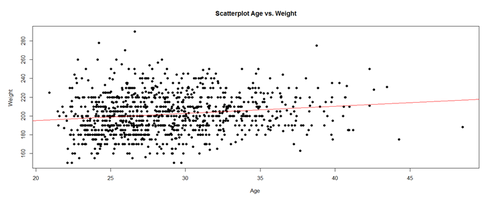Note that Age is different from the random effects for Team and Position, where the relationship between these and Weight is more unpredictable or stochastic. Look at the residuals of lmer.model.2 output: summary(lmer.model.2) Linear mixed model fit by REML ['lmerMod'] Formula: Weight ~ Height + Age + (1 | Team) + (1 | Position) Data: data REML criterion at convergence: 8792.2 Scaled Residuals  Min 1Q Median 3Q Max -2.9434 -0.6454 -0.0414 0.6022 4.4715 Random Effects  Groups Name Variance Std. Dev. Team (Intercept) 1.136 1.066 Position (Intercept) 47.935 6.924 Residual 281.805 16.787 Number of obs: 1034, groups: Team, 30; Position, 9 Fixed Effects  Estimate Std. Error t Value (Intercept) -176.4537 19.0913 -9.243 Height 4.8043 0.2512 19.125 Age 0.8888 0.1222 7.273 Correlation of Fixed Effects  (Intr) Height Height -0.974 Age -0.240 0.056 Note that compared to our earlier model without the fixed effect for Age, the variation that’s associated with the random effects for “Team” and “Position” are reduced. This is because the variation due to Age may be confounded with the variation that’s due to Team/Position. As the initial model didn’t know about Age, its predictions were relatively more varying, producing larger residuals. Exploring the fixed effect coefficients: Fixed Effects  Estimate Std. Error t Value (Intercept) -176.4537 19.0913 -9.243 Height 4.8043 0.2512 19.125 Age 0.8888 0.1222 7.273 We see that the impact of Age on Weight is about 0.9, with an intercept of -176 (pounds). The coefficient for the fixed effect of Height changed slightly, from 4.6973 to 4.8. ### Scientific Inference Reporting LME models requires explicit quantitative estimation of probability values expressing the statistical significance of these estimates. Interpretation of p-values for mixed models is different from their counterparts in fixed-effects linear models. The Likelihood Ratio Test provides one approach to obtain the p-values. Likelihood is the (conditional) probability of seeing the observed data given a model. The likelihood ratio test compares the (ratio of) two likelihoods corresponding to two alternative models. For instance, suppose we are interested in quantifying the significance of a factor A. The model without the factor A (the null model), will be compared to the model with the factor A. Suppose we are comparing the following 2 models aiming to assess the significance of the impact of Age on Weight. m1: Weight ~ Height + Age m2: Weight ~ Height A significant difference between “m2” and “m1” would yield that Age matters as a predictor of Weight. Similarly, to estimate the effect of the Height, you would have to do a similar comparison: m1’: Weight ~ Height + Age m2’: Weight ~ Age In both cases, we compared a full model (with the fixed effects in question) against a reduced model without the variable of interest explicitly modeled. A fixed effect would be significant if the ratio between the likelihoods of these two models is significant. In R, we start by constructing the null model: lmer.model.0 <- lmer(Weight ~ Height + (1|Team) + (1|Position), data=data, REML=FALSE) Note the added the argument REML=FALSE that changes the calculation of the likelihood estimator, which is required when comparing models using the likelihood ratio test. Then, we re-do the full model, also with REML=FALSE: lmer.model.1 <- lmer(Weight ~ Height + Age+ (1|Team) + (1|Position), data=data, REML=FALSE) To compare the two models (the full model with the effect in question with the null model without the effect), we perform the likelihood ratio test using the anova() function: anova(lmer.model.0, lmer.model.1) The output of this call is: Data: data Models: lmer.model.0: Weight ~ Height + (1 | Team) + (1 | Position) lmer.model.1: Weight ~ Height + Age + (1 | Team) + (1 | Position) Df AIC BIC logLik deviance Chisq Chi Df Pr(>Chisq) lmer.model.0 5 8854.3 8879.0 -4422.1 8844.3 lmer.model.1 6 8804.5 8834.1 -4396.2 8792.5 51.795 1 6.16e-13 *** --- Signif. codes: 0 ‘***’ 0.001 ‘**’ 0.01 ‘*’ 0.05 ‘.’ 0.1 ‘ ’ 1  The report starts with the formulas of the two models, computes a Chi-Square statistics with associated degrees of freedom (2-1), and reports the p-value representing the strength of the data-driven evidence to reject the null-model (and hence to accept the significance of the factor of interest, Age, as impactful on Weight). The qualitative interpretation of these quantitative results may be stated as: “… Age affects Weight X2(1)=51.79, p< 10-12), increasing it (annually) by about 0.89 lb ± 0.1222 (standard error) …” We are using a Chi-Square test because Wilk’s Theorem states that$-2log(\frac{Likelihood\_Null\_model(m0)}{Likelihood\_full\_model(m1)}$) approaches a Chi-Square distribution with degrees of freedom equal to the number of parameters that differ between the models (in this case, only “Age”, so df=1). This likelihood-based inference approach is somewhat different from the classical t-tests, ANOVA and linear model inference. Instead of obtaining a p-value directly from the MLE model, we need to run a second-order analysis (in this case ANOVA on the 2 models) to compare them and derive a p-value. Note that the “Height” predictor was present in both models (null and full models) as we aimed to assess the impact of Age using the likelihood ratio test. In this test, we think of the covariate “Height” as a control variable and of “Age” as the test variable. What happens if we compare the following two models: m0: Weight ~ 1 # intercept only constant model estimating the mean only m1: Weight ~ Height + Age # Intercept and 2 covariates model Suppose the likelihood-ration test suggest significant differences between the 2 models, this would suggest that “m1” (full) and “m0” (null) are significantly different from one another. However, it would not attribute this difference to “Height” or “Age” alone. We may not be able to tease out conclusively which one of the two variables (or both) was crucial. How about the possibility of having an Age-by-Height interaction effect? For instance, “Height” effect on Weight may be modulated through “Age”. If such inter-dependence between two factors (an interaction) is present or suspected, its impact may be assessed the same way: full model: Weight ~ Height * Age reduced model: Weight ~ Height + Age Interactions between two factors are commonly specified with a “*” rather than a “+”. Comparing these models via the likelihood ratio test and the anova() function yields a p-value quantifying the significance of the interaction term (Height * Age). A significant result implies that Height and Age are strongly inter-dependent on each other. An insignificant result implies that there is no strong evidence in these data for inter-dependence. Note: lm.1 <- lm(y ~ x:z) # includes only the interaction (colon, “:”) between x & z, # but not the original variables x and z lm.2 <- lm(y ~ x + z + x:z) # this is a complete model for y using x & z #In regressions with a lot of variables, the product (*) notation provides a shortcut to minimize typing. # We can indicate this using a simple multiplication symbol (*): lm.3 <- lm(y ~ x*z) # equivalent to model lm.2, but shorter. Using this code, R will automatically include both variables in the regression in addition to the interaction between the two We can experiment with computing alternative likelihoods to contrast different models using these, or other, datasets. For instance, compare “Height*Age” vs. “Height + Age” vs. simply “1” (intercept only model). We need to specify REML=FALSE in the models to be able to use the log-likelihood ratio test. Interpreting effect-sizes for random effects (random slopes vs. random intercepts) Let’s inspect the coefficients of the mixed model by Team and by Position: coef(lmer.model.1) $\Team {| class="wikitable" style="text-align:center; width:35%" border="1" |||(Intercept)||Height||Age |- |ANA||-176.9872||4.810485||0.8900941 |- |ARZ||-176.2895||4.810485||0.8900941 |- |ATL||-177.2084||4.810485||0.8900941 |- |BAL||-177.6145||4.810485||0.8900941 |- |BOS||-177.0276||4.810485||0.8900941 |- |CHC||-176.9496||4.810485||0.8900941 |- |CIN||-176.7425||4.810485||0.8900941 |- |CLE||-177.1519||4.810485||0.8900941 |- |COL||-177.4466||4.810485||0.8900941 |- |CWS||-176.4970||4.810485||0.8900941 |- |DET||-176.7084||4.810485||0.8900941 |- |FLA||-176.7071||4.810485||0.8900941 |- |HOU||-177.0758||4.810485||0.8900941 |- |KC||-177.5616||4.810485||0.8900941 |- |LA||-176.6696||4.810485||0.8900941 |- |MIN||-176.7251||4.810485||0.8900941 |- |MLW||-176.4952||4.810485||0.8900941 |- |NYM||-177.2486||4.810485||0.8900941 |- |NYY||-176.7923||4.810485||0.8900941 |- |OAK||-177.0576||4.810485||0.8900941 |- |PHI||-177.8202||4.810485||0.8900941 |- |PIT||-176.4746||4.810485||0.8900941 |- |SD||-176.6908||4.810485||0.8900941 |- |SEA||-177.1092||4.810485||0.8900941 |- |SF||-176.8983||4.810485||0.8900941 |- |STL||-177.1223||4.810485||0.8900941 |- |TB||-177.2853||4.810485||0.8900941 |- |TEX||-177.0813||4.810485||0.8900941 |- |TOR||-176.8177||4.810485||0.8900941 |- |WAS||-177.2275||4.810485||0.8900941 |} </center> <center> \Position

 (Intercept) Height Age Catcher -172.2235 4.810485 0.8900941 Designated_Hitter -166.9709 4.810485 0.8900941 First_Baseman -169.9896 4.810485 0.8900941 Outfielder -177.8433 4.810485 0.8900941 Relief_Pitcher -179.5896 4.810485 0.8900941 Second_Baseman -183.9341 4.810485 0.8900941 Shortstop -186.9628 4.810485 0.8900941 Starting_Pitcher -179.2067 4.810485 0.8900941 Third_Baseman -176.1245 4.810485 0.8900941
attr(,"class")
 "coef.mer"


As expected from a mixed-effect model, each Team and each Position is assigned a different intercept, as we specified the model with “(1|Team)” and “(1|Position)” to take into account by-Team and by-Position variability:

lmer.model.1 <- lmer(Weight ~ Height + Age + (1|Team) + (1|Position), data=data, REML=FALSE)

The fixed effects (Height + Age) are all the same for all Team and Positions, as this model is a random intercept model where we account for baseline-differences in Weight assuming whatever the effect of Height is, it’s the same for all Teams and Positions.

This assumption may not always be valid – some Positions may demand more or less Weight. The Weight effect may be different for different Positions. Similarly, the Weight effect may be different for different Teams (recall the Water Polo National teams – Spain vs. Serbia).

Thus, we may need a random slope model, where Team and Positions may have differing intercepts as well as different slopes for the Weight. In R this can be coded as:

lmer.model.2 = lmer(Weight ~ Height + Age + (1+Height|Team) + (1+Height|Position), data=data, REML=FALSE)

This new model only includes more complicated random effects. In this case, the notation “(1+Height|Team)” means that the model expects differing baseline-levels of Weight (the intercept, represented by 1) and differing levels of “Height”. The same is true for Position.

Examining the coefficients of this updated model:

coef(lmer.model.2)


Generates the following output:

\$\Team {| class="wikitable" style="text-align:center; width:35%" border="1" |- | ||(Intercept)||Height||Age |- |ANA||-184.5752||4.913632||0.8799488 |- |ARZ||-157.6363||4.564806||0.8799488 |- |ATL||-185.3436||4.923583||0.8799488 |- |BAL||-193.6560||5.031218||0.8799488 |- |BOS||-183.8410||4.904126||0.8799488 |- |CHC||-181.9705||4.879904||0.8799488 |- |CIN||-176.1931||4.805094||0.8799488 |- |CLE||-195.5968||5.056349||0.8799488 |- |COL||-193.8297||5.033467||0.8799488 |- |CWS||-174.9203||4.788613||0.8799488 |- |DET||-168.4951||4.705414||0.8799488 |- |FLA||-180.6757||4.863139||0.8799488 |- |HOU||-191.5622||5.004105||0.8799488 |- |KC||-204.9527||5.177497||0.8799488 |- |LA||-166.8079||4.683568||0.8799488 |- |MIN||-182.1954||4.882816||0.8799488 |- |MLW||-164.4339||4.652826||0.8799488 |- |NYM||-184.1068||4.907567||0.8799488 |- |NYY||-186.9822||4.944800||0.8799488 |- |OAK||-184.9647||4.918675||0.8799488 |- |PHI||-208.3698||5.221744||0.8799488 |- |PIT||-176.5423||4.809616||0.8799488 |- |SD||-169.8045||4.722369||0.8799488 |- |SEA||-183.9884||4.906034||0.8799488 |- |SF||-180.9398||4.866558||0.8799488 |- |STL||-183.5529||4.900395||0.8799488 |- |TB||-186.5983||4.939829||0.8799488 |- |TEX||-182.5549||4.887472||0.8799488 |- |TOR||-193.1088||5.024132||0.8799488 |- |WAS||-203.4900||5.158557||0.8799488 |} </center> <center> \Position

 (Intercept) Height Age Catcher -209.6130 5.323756 0.8799488 Designated_Hitter -236.8530 5.766876 0.8799488 First_Baseman -219.8348 5.490036 0.8799488 Outfielder -180.9668 4.857761 0.8799488 Relief_Pitcher -172.6381 4.722276 0.8799488 Second_Baseman -141.1982 4.210837 0.8799488 Shortstop -127.4526 3.987233 0.8799488 Starting_Pitcher -173.4682 4.735780 0.8799488 Third_Baseman -191.4820 5.028815 0.8799488
attr(,"class")
 "coef.mer"


Note that the columns containing the by-team and by-position coefficients for the effect of “Height” are different for each Team and each Position. The fact that this column's values are always positive (quite similar to one another) implies that despite variation between teams/positions there is consistency in how Height impacts Weight. In other words, the Weight of all Players tends to go UP with Height, which is to be expected, albeit for some positions Weight may go UP slightly more than for other positions (e.g., Designated_Hitter=5.766876 vs. Shortstop=3.987233). On the other hand, the coefficients for Age remain static across positions and teams – why? Because the model (lmer.model.2) didn’t specify random slopes for the by-Position or by-Team effect of Age.

Next, we need to address the significance of these effects (i.e., compute the corresponding p-values using the likelihood ration test). We keep our model from above (lmer.model.2) and compare it to a new null model (lmer.model.0) using the likelihood ratio test:

lmer.model.0 = lmer(Weight ~ Age + (1+Height|Team) + (1+Height|Position), data=data, REML=FALSE)

lmer.model.2 = lmer(Weight ~ Height + Age + (1+Height|Team) + (1+Height|Position), data=data, REML=FALSE)

The null model (lmer.model.0) needs to have the same random effects structure as the full model (lmer.model.2). That is, all random slope parameters included in the full model must be present in the null model.

Let’s now do the likelihood ratio test:

anova(lmer.model.0, lmer.model.2)

Models:
lmer.model.0: Weight ~ Age + (1 + Height | Team) + (1 + Height | Position)
lmer.model.2: Weight ~ Height + Age + (1 + Height | Team) + (1 + Height | Position)
Df    AIC    BIC  logLik deviance  Chisq Chi Df Pr(>Chisq)
lmer.model.0  9 8876.4 8920.8 -4429.2   8858.4
lmer.model.2 10 8809.3 8858.7 -4394.6   8789.3 69.082      1  < 2.2e-16 ***
---
Signif. codes:  0 ‘***’ 0.001 ‘**’ 0.01 ‘*’ 0.05 ‘.’ 0.1 ‘ ’ 1
`

Model differences are statistically significant. In general, ask: do we need random effects? and if so, which random slopes to include in the model? It’s a bit easier to work with random effect models that only include random intercept. However, it may be best to always include the random slopes in the models, as if these are insignificant, so we can determine that and reduce the model. In general, we can expect that the effect of an experimental manipulation (e.g., player position, team, etc.) may not be static across items (e.g., locations). There is some evidence from simulation studies that mixed models excluding random slopes may be too liberal - their Type I error rate may be higher than expected (i.e., we can find significant effects which are actually due to chance alone).

The full model (lmer.model.2) is focused on baseball players’ Weight. As Age is not a variable we are interested in studying, albeit it may affect Weight, we need to control for Age. Thus lmer.model.2 has random slopes for the effect of Height (by Team and Position) but not the effect of Age. In other words, we only modeled by-Team and by-Position variability in how Height affects Weight.

lmer.model.2 = lmer(Weight ~ Height + Age + (1+Height|Team) + (1+Height|Position), data=data, REML=FALSE)